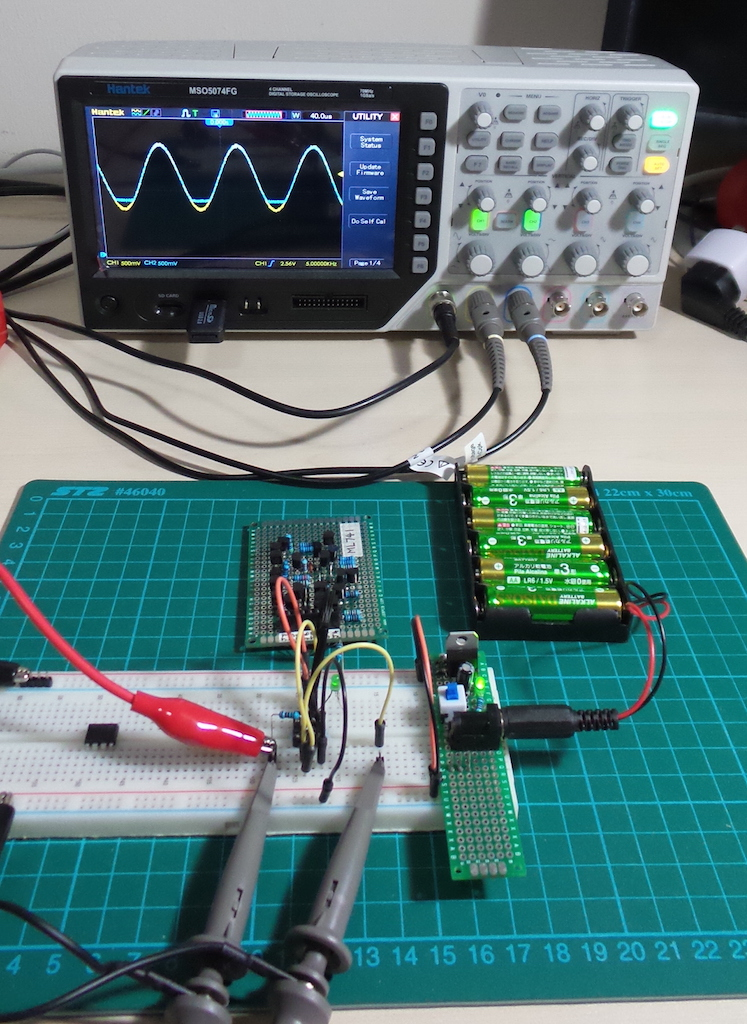# #157 ML741/VoltageFollower

Test a voltage follower/buffer circuit with the ML741 discrete component opamp

This is a demonstration of a voltage follower circuit using the ML741 discrete component opamp. The general operation of a voltage follower is for the output to follow the non-inverting input, with a gain of 1, i.e.

``````Vout = Vin
``````### How it works

Fundamentally, an op-amp strives to keep its inverting an non-inverting inputs equal by modulating the output.

When the non-inverting input rises above the inverting input, the output will rise to offset the differential. Since there is 100% feedback to the non-inverting input, the output will immediately change to match the inverting input.

Voltage followers, also known as unity gain buffers, are often used to isolate circuit sub-systems since they offer - at least in the idealised op-amp model - infinite impedence at the input and zero impedance at the output.

## Construction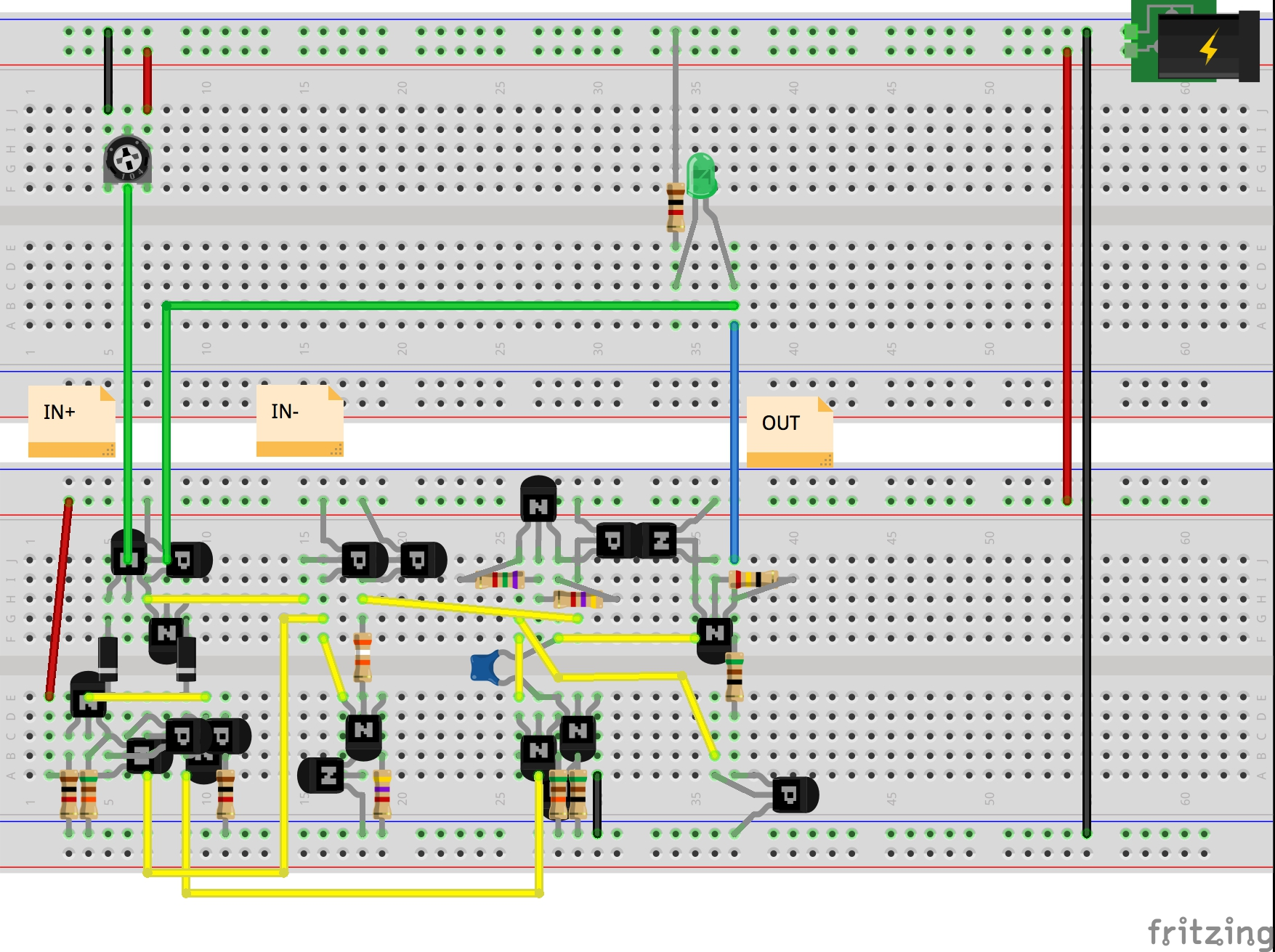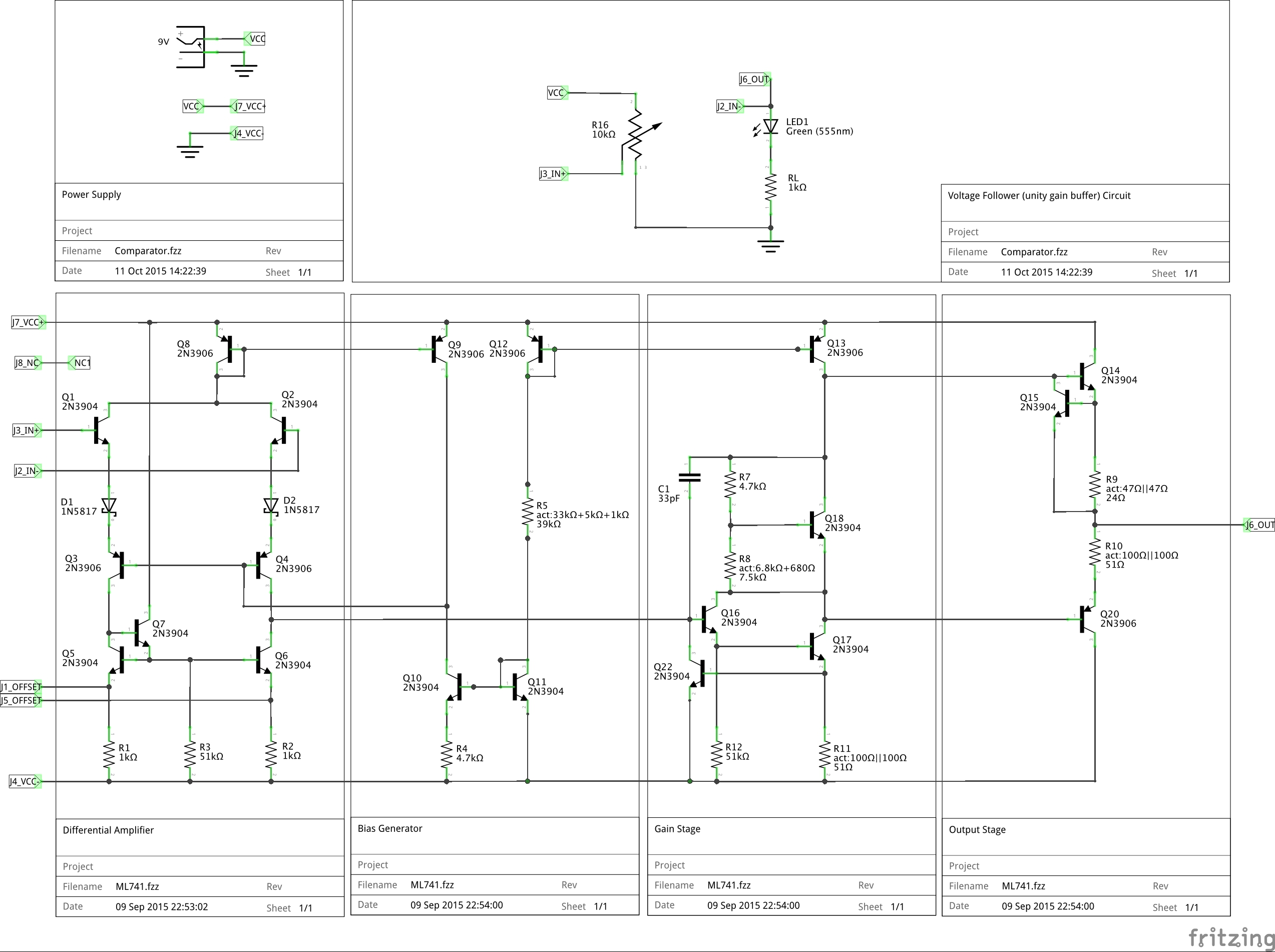ML741 on the right, and a standard UA741CN on the left waiting to be put to the test..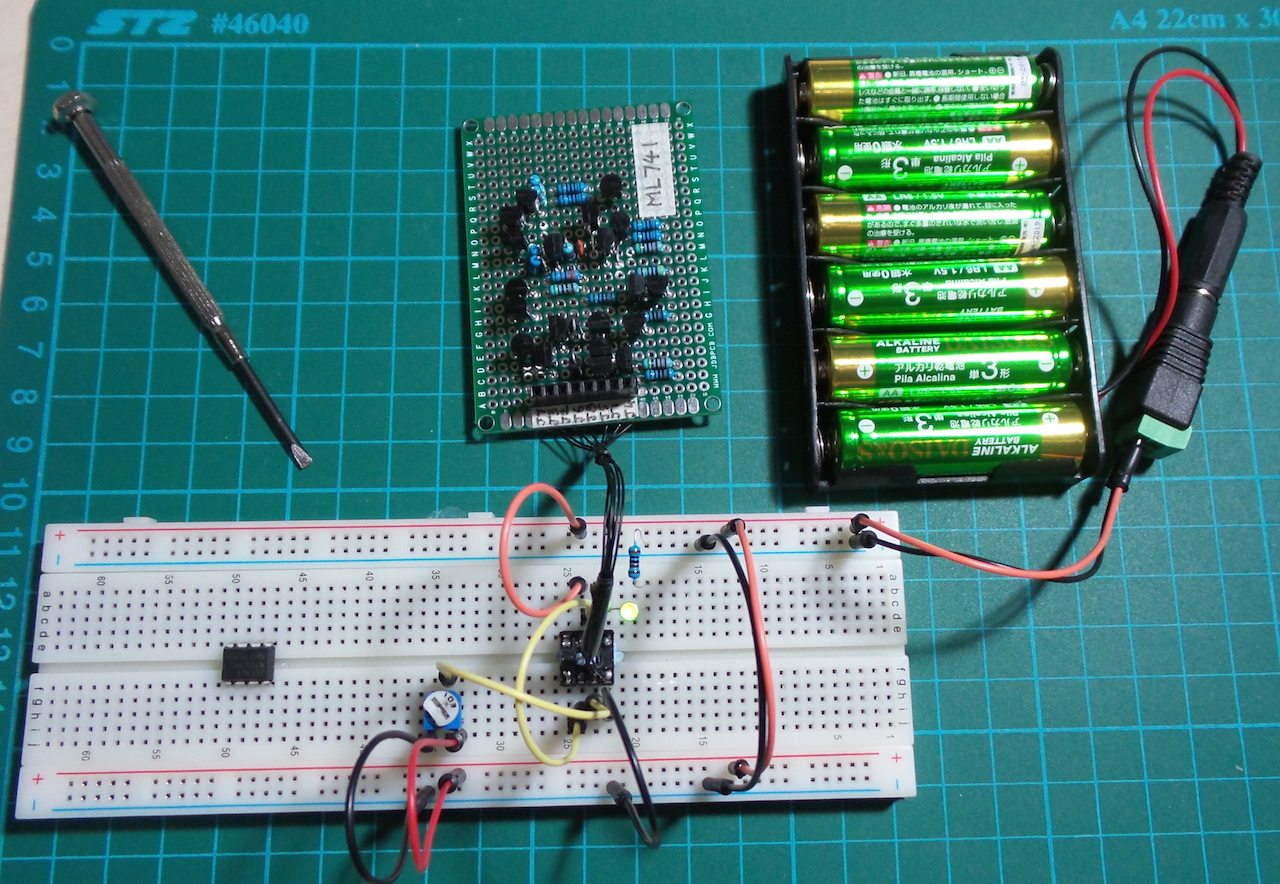## ML741 v “real” 741 Test

Here are some results comparing the behaviour of a standard UA741CN chip with the ML741 (protoboard version).

Setup:

• power is 5V single rail, i.e. V- = GND
• non-inverting input is fed a sine wave 1Vpp with 2.5V DC offset (with a 1kΩ resistor in series for redundant protection)
• the function generator sine wave input replaces the manual 10kΩ pot in the schematic above

Scope connections

• CH1: non-inverting input
• CH2: output/inverting input

I’ve purposely driven the input signal such that it clips the lower output rail of both the ML741 and UA741CN, because things get interesting around the rails.

### At 20kHz

• both tracking the input voltage quite well
• clean clipping at the lower output rail
• ML741 again proves its ability to drive lower than the standard UA741CN

ML741: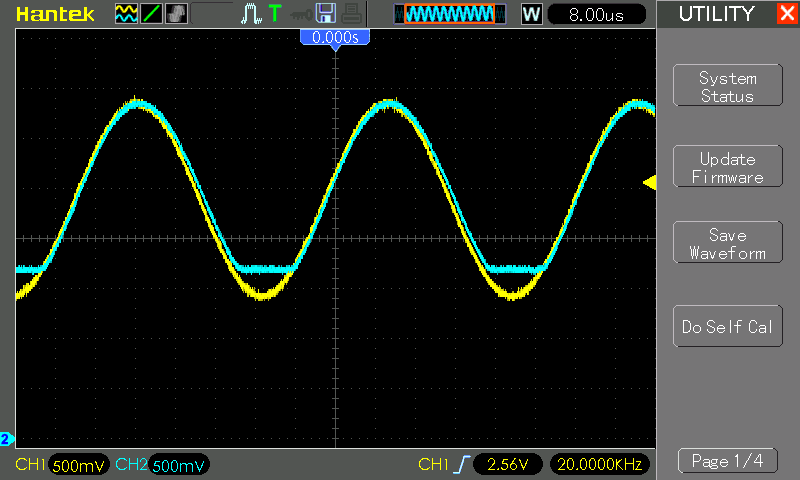UA741CN: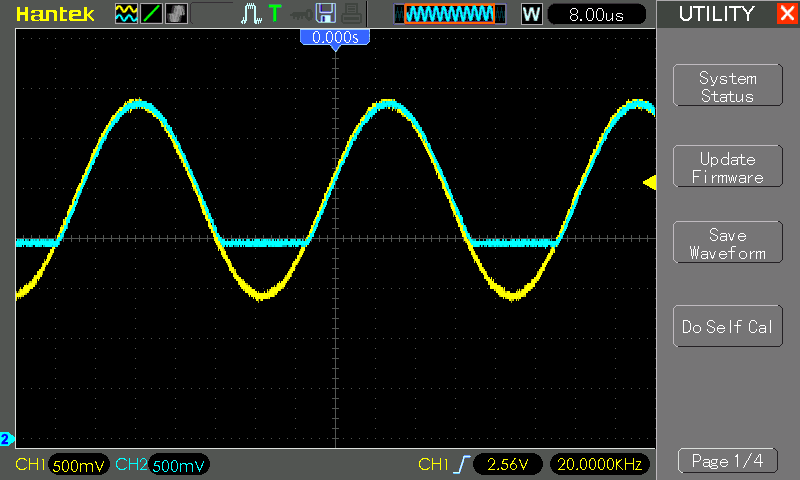### At 80kHz

• both struggling to drop the output voltage fast enough
• the poorer ML741 response time has effectively increased its lower output limit so it is now higher than the UA741CN
• ML741 is the first to get into trouble (from 40kHz at least)

ML741: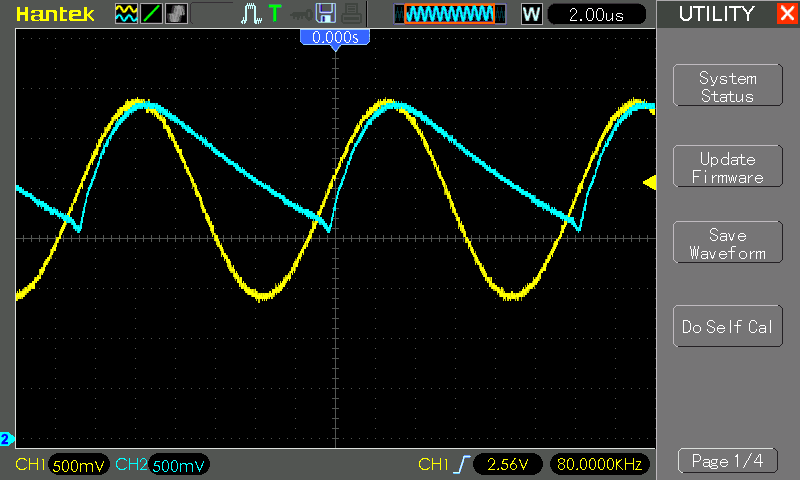UA741CN: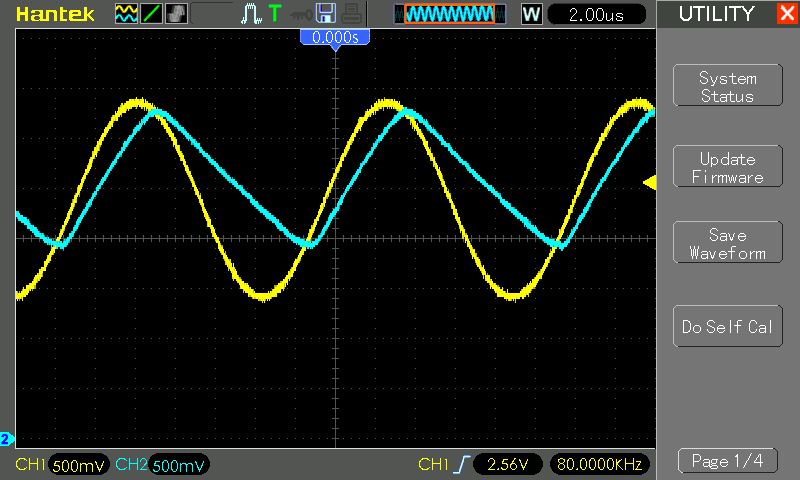Measurements in action…